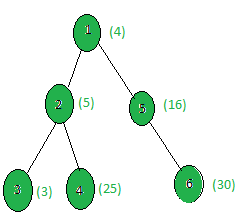# Count the nodes whose weight is a perfect square

Given a tree, and the weights of all the nodes, the task is to count the number of nodes whose weight is a perfect Square.

Examples:

Input:Output: 3
Only the weights of nodes 1, 4 and 5 are perfect squares.

## Recommended: Please try your approach on {IDE} first, before moving on to the solution.

Approach: Perform dfs on the tree and for every node, check if it’s weight is a perfect square or not.

Below is the implementation of the above approach:

## C++

 `// C++ implementation of the approach ` `#include ` `using` `namespace` `std; ` ` `  `int` `ans = 0; ` ` `  `vector<``int``> graph; ` `vector<``int``> weight(100); ` ` `  `// Function that returns true ` `// if n is a perfect square ` `bool` `isPerfectSquare(``int` `n) ` `{ ` `    ``double` `x = ``sqrt``(n); ` `    ``if` `(``floor``(x) != ``ceil``(x)) ` `        ``return` `false``; ` `    ``return` `true``; ` `} ` ` `  `// Function to perform dfs ` `void` `dfs(``int` `node, ``int` `parent) ` `{ ` `    ``// If weight of the current node ` `    ``// is a perfect square ` `    ``if` `(isPerfectSquare(weight[node])) ` `        ``ans += 1; ` ` `  `    ``for` `(``int` `to : graph[node]) { ` `        ``if` `(to == parent) ` `            ``continue``; ` `        ``dfs(to, node); ` `    ``} ` `} ` ` `  `// Driver code ` `int` `main() ` `{ ` `    ``int` `x = 15; ` ` `  `    ``// Weights of the node ` `    ``weight = 4; ` `    ``weight = 5; ` `    ``weight = 3; ` `    ``weight = 25; ` `    ``weight = 16; ` `    ``weight = 30; ` ` `  `    ``// Edges of the tree ` `    ``graph.push_back(2); ` `    ``graph.push_back(3); ` `    ``graph.push_back(4); ` `    ``graph.push_back(5); ` `    ``graph.push_back(6); ` ` `  `    ``dfs(1, 1); ` ` `  `    ``cout << ans; ` ` `  `    ``return` `0; ` `} `

## Java

 `// Java implementation of the approach ` `import` `java.util.*; ` ` `  `class` `GFG{ ` `  `  `static` `int` `ans = ``0``; ` `  `  `static` `Vector[] graph = ``new` `Vector[``100``];  ` `static` `int``[] weight = ``new` `int``[``100``]; ` `  `  `// Function that returns true ` `// if n is a perfect square ` `static` `boolean` `isPerfectSquare(``int` `n) ` `{ ` `    ``double` `x = Math.sqrt(n); ` `    ``if` `(Math.floor(x) != Math.ceil(x)) ` `        ``return` `false``; ` `    ``return` `true``; ` `} ` `  `  `// Function to perform dfs ` `static` `void` `dfs(``int` `node, ``int` `parent) ` `{ ` `    ``// If weight of the current node ` `    ``// is a perfect square ` `    ``if` `(isPerfectSquare(weight[node])) ` `        ``ans += ``1``; ` `  `  `    ``for` `(``int` `to : graph[node]) { ` `        ``if` `(to == parent) ` `            ``continue``; ` `        ``dfs(to, node); ` `    ``} ` `} ` `  `  `// Driver code ` `public` `static` `void` `main(String[] args) ` `{ ` `    ``int` `x = ``15``; ` `    ``for` `(``int` `i = ``0``; i < ``100``; i++)  ` `        ``graph[i] = ``new` `Vector<>(); ` `     `  `    ``// Weights of the node ` `    ``weight[``1``] = ``4``; ` `    ``weight[``2``] = ``5``; ` `    ``weight[``3``] = ``3``; ` `    ``weight[``4``] = ``25``; ` `    ``weight[``5``] = ``16``; ` `    ``weight[``6``] = ``30``; ` `  `  `    ``// Edges of the tree ` `    ``graph[``1``].add(``2``); ` `    ``graph[``2``].add(``3``); ` `    ``graph[``2``].add(``4``); ` `    ``graph[``1``].add(``5``); ` `    ``graph[``5``].add(``6``); ` `  `  `    ``dfs(``1``, ``1``); ` `  `  `    ``System.out.print(ans);  ` `} ` `} ` ` `  `// This code is contributed by Rajput-Ji `

## Python3

 `# Python3 implementation of the approach ` `from` `math ``import` `*` `ans ``=` `0` ` `  `graph ``=` `[[] ``for` `i ``in` `range``(``100``)] ` `weight ``=` `[``0``] ``*` `100` ` `  `# Function that returns true ` `# if n is a perfect square ` `def` `isPerfectSquare(n): ` `    ``x ``=` `sqrt(n) ` `    ``if` `(floor(x) !``=` `ceil(x)): ` `        ``return` `False` `    ``return` `True` ` `  `# Function to perform dfs ` `def` `dfs(node, parent): ` `    ``global` `ans ` `     `  `    ``# If weight of the current node  ` `    ``# is a perfect square ` `    ``if` `(isPerfectSquare(weight[node])): ` `        ``ans ``+``=` `1``; ` `     `  `    ``for` `to ``in` `graph[node]: ` `        ``if` `(to ``=``=` `parent): ` `            ``continue` `        ``dfs(to, node) ` ` `  `# Driver code ` ` `  `x ``=` `15` ` `  `# Weights of the node ` `weight[``1``] ``=` `4` `weight[``2``] ``=` `5` `weight[``3``] ``=` `3` `weight[``4``] ``=` `25` `weight[``5``] ``=` `16` `weight[``6``] ``=` `30` ` `  `# Edges of the tree ` `graph[``1``].append(``2``) ` `graph[``2``].append(``3``) ` `graph[``2``].append(``4``) ` `graph[``1``].append(``5``) ` `graph[``5``].append(``6``) ` ` `  `dfs(``1``, ``1``) ` `print``(ans) ` ` `  `# This code is contributed by SHUBHAMSINGH10 `

Output:

```3
```

Complexity Analysis:

• Time Complexity: O(N*logV) where V is the maximum weight of a node in the tree.
In DFS, every node of the tree is processed once and hence the complexity due to the DFS is O(N) for N nodes in the tree. Also, while processing every node, in order to check if the node value is a perfect square or not, the inbuilt sqrt(V), is being called where V is the weight of the node and this function has a complexity of O(log V). Hence for every node, there is an added complexity of O(log V). Therefore, the total time complexity is O(N*logV).
• Auxiliary Space: O(1).
Any extra space is not required, so the space complexity is constant.

Attention reader! Don’t stop learning now. Get hold of all the important DSA concepts with the DSA Self Paced Course at a student-friendly price and become industry ready.

My Personal Notes arrow_drop_upCheck out this Author's contributed articles.

If you like GeeksforGeeks and would like to contribute, you can also write an article using contribute.geeksforgeeks.org or mail your article to contribute@geeksforgeeks.org. See your article appearing on the GeeksforGeeks main page and help other Geeks.

Please Improve this article if you find anything incorrect by clicking on the "Improve Article" button below.

Article Tags :
Practice Tags :

Be the First to upvote.

Please write to us at contribute@geeksforgeeks.org to report any issue with the above content.